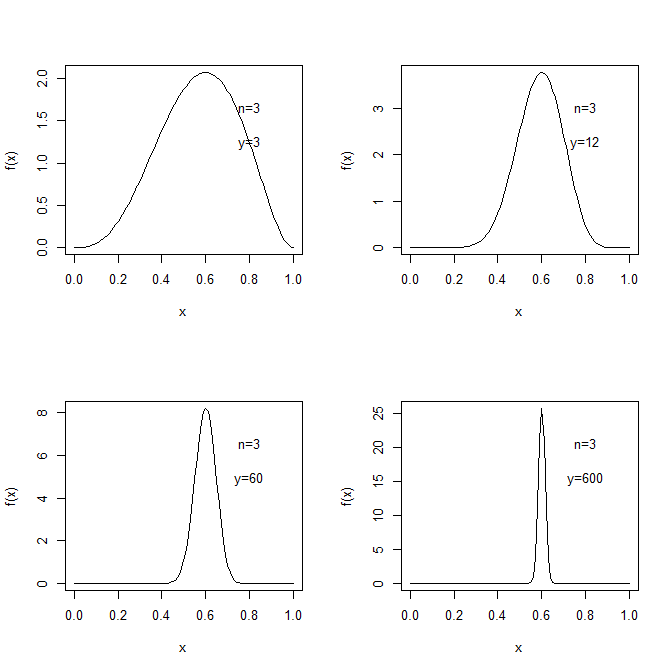BMSI系列的第一篇，介绍了贝叶斯框架下的单参数模型，同时也算是贝叶斯入门的第一步。

## 一个简单的引入——二项分布

### 贝叶斯方法

$p(\theta,y)=p(\theta)p(y\lvert \theta)=p(y)p(\theta\lvert y)$

$p(\theta\lvert y)=p(\theta)p(y\lvert \theta)/p(y)\propto p(\theta)p(y\lvert \theta)$

$p(y\lvert \theta)=\binom{n}{y}\theta^y (1-\theta)^{n-y}$

$p(\theta\lvert y)\propto \theta^y (1-\theta)^{n-y}$

$\theta\lvert y\sim\mathrm{Beta}(y+1,n-y+1)$$\mathbb{E}(\theta\lvert y)=\frac{\alpha}{\alpha+\beta}=\frac{y+1}{n+2}$

$\frac{y+1}{n+2}=\frac{n}{n+2}\frac{y}{n}+\frac{2}{n+2}\frac{1}{2}$

## 后验分布的性质

$\mathbb{E}(\theta)=\mathbb{E}(\mathbb{E}(\theta\lvert y))$ $\mathrm{Var}(\theta)=\mathbb{E}(\mathrm{Var}(\theta\lvert y))+\mathrm{Var}(\mathbb{E}(\theta\lvert y))$

• 第一条说明：先验分布的均值$\mathbb{E}(\theta)$是所有后验分布的均值$\mathbb{E}(\theta\lvert y)$在数据分布$p(y)$上的加权平均。

• 第二条说明：后验分布的方差$\mathrm{Var}(\theta\lvert y)$在平均意义上(数据分布$p(y)$上的加权平均)不会超过先验方差$\mathrm{Var}(\theta)$
• 其直观理解是，输入了信息不会使得我们的预测的方差更大
• 值得注意的是，其只保证了平均意义上后验方差不会更大。对于某一具体的观测$y$，其后验方差是可以超过先验方差的。
• 第二条还说明：后验均值的方差越大，$\theta$的方差减少（对比先后验）就更多。

## 先验的选择

### 共轭先验

#### 优劣

• 先验和后验有相同的形式：因为均属于同一个分布族。这通常意味着我们能给出一个闭式解
• 先验和后验有相同的形式，意味着我们可以将后验作为下一时刻的先验：因此我们可以不断根据新来的数据然后调整后验分布的参数，也就是所谓的在线贝叶斯学习(online bayesian learning)
• 通常，我们可以将先验解释为额外的数据，这使得我们的模型有良好的解释性

• 实际应用中，复杂模型可能无法求出共轭先验
• 使用非共轭先验并没有带来任何的问题
• 许多非共轭先验其实是使用共轭先验的混合构造出来的，因为单纯的共轭先验可能并不合理

#### 求法

$p(y_i\lvert\theta)=f(y_i)g(\theta)\exp\{\phi(\theta)^\intercal u(y_i)\}$

$p(y\lvert\theta)=\left(\prod_{i=1}^{n}f(y_i)\right)g(\theta)^n\exp\{\phi(\theta)^\intercal \sum_{i=1}^nu(y_i)\}\propto g(\theta)^n\exp\{\phi(\theta)^\intercal t(y)\}$

$p(\theta\lvert y)\propto g(\theta)^{\eta+n}\exp\{\phi(\theta)^\intercal (\nu+t(y))\}$

#### 例子

##### 已知方差的正态分布

$p(\theta\lvert y)\propto\exp\left\{-\frac{1}{2}\left(\frac{(y-\theta)^2}{\sigma^2}+\frac{(\theta-\mu_0)^2}{\tau_0^2}\right)\right\}$

$\mu_1=\frac{\frac{\mu_0}{\tau_0^2}+\frac{y}{\sigma^2}}{\frac{1}{\tau_0^2}+\frac{1}{\sigma^2}}\quad\mathrm{and}\quad\frac{1}{\tau_1^2}=\frac{1}{\tau_0^2}+\frac{1}{\sigma^2}$

$p(\tilde{y}\lvert y)=\int p(\tilde{y}\lvert \theta)p(\theta\lvert y)\,\mathrm{d}\theta\propto\int \exp\left\{-\frac{(\tilde{y}-\theta)^2}{2\sigma^2}\right\}\exp\left\{-\frac{(\theta-\mu_1)^2}{2\tau_1^2}\right\}\,\mathrm{d}\theta$

$\mathbb{E}(\tilde{y}\lvert y)=\mathbb{E}(\mathbb{E}(\tilde{y}\lvert \theta,y)\lvert y)=\mathbb{E}(\theta\lvert y)=\mu_1$

$\mathrm{Var}(\tilde{y}\lvert y)=\mathbb{E}(\mathrm{Var}(\tilde{y}\lvert y)\lvert y)+\mathrm{Var}(\mathbb{E}(\theta\lvert y)\lvert y)=\mathbb{E}(\sigma^2\lvert y)+\mathrm{Var}(\theta\lvert y)=\sigma^2+\tau_1^2$

$\mu_n=\frac{\frac{1}{\tau_0^2}\mu_0+\frac{n}{\sigma^2}\bar{y}}{\frac{1}{\tau_0^2}+\frac{n}{\sigma^2}}\quad\mathrm{and}\quad\frac{1}{\tau_n^2}=\frac{1}{\tau_0^2}+\frac{n}{\sigma^2}$

##### 已知均值的正态分布

$p(y\lvert\sigma^2)\propto \sigma^{-n}\exp\left\{-\frac{1}{2\sigma^2}\sum_{i=1}^n(y_i-\theta)^2\right\}\overset{\Delta}{=}(\sigma^2)^{-n/2}\exp\left\{\frac{-n\nu}{2\sigma^2}\right\}$

$p(\sigma^2)\propto(\sigma^2)^{-(\nu_0/2+1)}\exp\left\{-\frac{\nu_0\sigma_0^2}{2\sigma^2}\right\}$

\begin{align} p(\sigma^2\lvert y) & \propto p(\sigma^2)p(y\lvert\sigma^2)\\ & \propto (\sigma^2)^{-(\nu_0/2+1)}\exp\left\{-\frac{\nu_0\sigma_0^2}{2\sigma^2}\right\}(\sigma^2)^{-n/2}\exp\left\{\frac{-n\nu}{2\sigma^2}\right\}\\ & \propto (\sigma^2)^{-((n+\nu_0)/2+1)}\exp\left\{-\frac{\nu_0\sigma_0^2+n\nu}{2\sigma^2}\right\} \end{align}

$\sigma^2\lvert y\sim \mathrm{Inv-}\chi^2((n+\nu_0),(\nu_0\sigma_0^2+n\nu)/(\nu_0+n))$

##### 泊松分布

$p(y\lvert\sigma^2)=\prod_{i=1}^{n}\frac{\theta^{y_i}}{y_i!}\exp\{-\theta\}\propto \theta^{t(y)}\exp\{n\theta\},\,t(y)=\sum_{i=1}^n y_i=n\bar{y}$

$p(\theta)\propto \theta^{\alpha-1}\exp\{\beta\theta\}$

$p(\theta)\propto \theta^{\alpha+n\bar{y}-1}\exp\{\beta\theta+n\theta\},\,\theta\lvert y\sim\mathrm{Gamma}(\alpha+n\bar{y},\beta+n)$

### 无信息先验

#### 合适的先验

$\mu_1=\frac{\frac{\mu_0}{\tau_0^2}+\frac{y}{\sigma^2}}{\frac{1}{\tau_0^2}+\frac{1}{\sigma^2}}\quad\mathrm{and}\quad\frac{1}{\tau_1^2}=\frac{1}{\tau_0^2}+\frac{1}{\sigma^2}$

#### 均匀先验的问题

$\theta$是一个随机变量并有概率密度函数$p(\theta)$，记$\phi=h(\theta)$是一个一一映射，此时我们去计算$\phi$的密度函数，有

$f(\phi)=p(\theta)\Bigg\lvert\frac{\mathrm{d}\theta}{\mathrm{d}\phi}\Bigg\rvert=p(\theta)\lvert h^\prime(\theta)\rvert^{-1}$

#### Jeffrey 先验

$J(\theta)=\mathbb{E}\left(\left(\frac{\mathrm{d}\log p(y\lvert\theta)}{\mathrm{d}\theta}\right)\big\rvert\theta\right)=-\mathbb{E}\left(\frac{\mathrm{d}^2\,\log p(y\lvert\theta)}{\mathrm{d}\theta^2}\big\rvert\theta\right)$

$J(\phi)=-\mathbb{E}\left(\frac{\mathrm{d}^2\,\log p(y\lvert\phi)}{\mathrm{d}\phi^2}\Bigg\rvert\phi\right)=-\mathbb{E}\left(\frac{\mathrm{d}^2\,\log p(y\lvert|theta=h^{-1}(\phi))}{\mathrm{d}\theta^2}\Bigg\lvert\frac{\mathrm{d}\theta}{\mathrm{d}\phi}\Bigg\rvert^2\Bigg\rvert\theta\right)=J(\theta)\Bigg\lvert\frac{\mathrm{d}\theta}{\mathrm{d}\phi}\Bigg\rvert^2$

$J(\phi)^{1/2}=J(\theta)^{1/2}\Bigg\lvert\frac{\mathrm{d}\theta}{\mathrm{d}\phi}\Bigg\rvert$

$p(\sigma)\propto \frac{1}{\sigma},\qquad p(\sigma^2)\propto \frac{1}{\sigma^2},\qquad p(\log \sigma^2)\propto p(\log \sigma)\propto 1$

• 使用无信息先验，则$p(\theta)=1$，即$\theta\sim\mathrm{Beta}(1,1)$
• 使用Jeffrey先验，则$p(\theta)\propto\theta^{-1/2}(1-\theta)^{-1/2}$，即$\theta\sim\mathrm{Beta}(1/2,1/2)$
• 使用自然参数的无信息先验，则$p(\mathrm{logit}(\theta))=1$，即$\theta\sim\mathrm{Beta}(0,0)$

#### 位置族参数和尺度族参数

$\pi(\sigma)=\pi^{\ast}(\sigma)$，且$\pi^{ast}(\sigma)=\lvert\frac{\mathrm{d}\theta}{\mathrm{d}\sigma}\rvert\pi(\theta)=\frac{1}{c}\pi(\frac{\sigma}{c})$